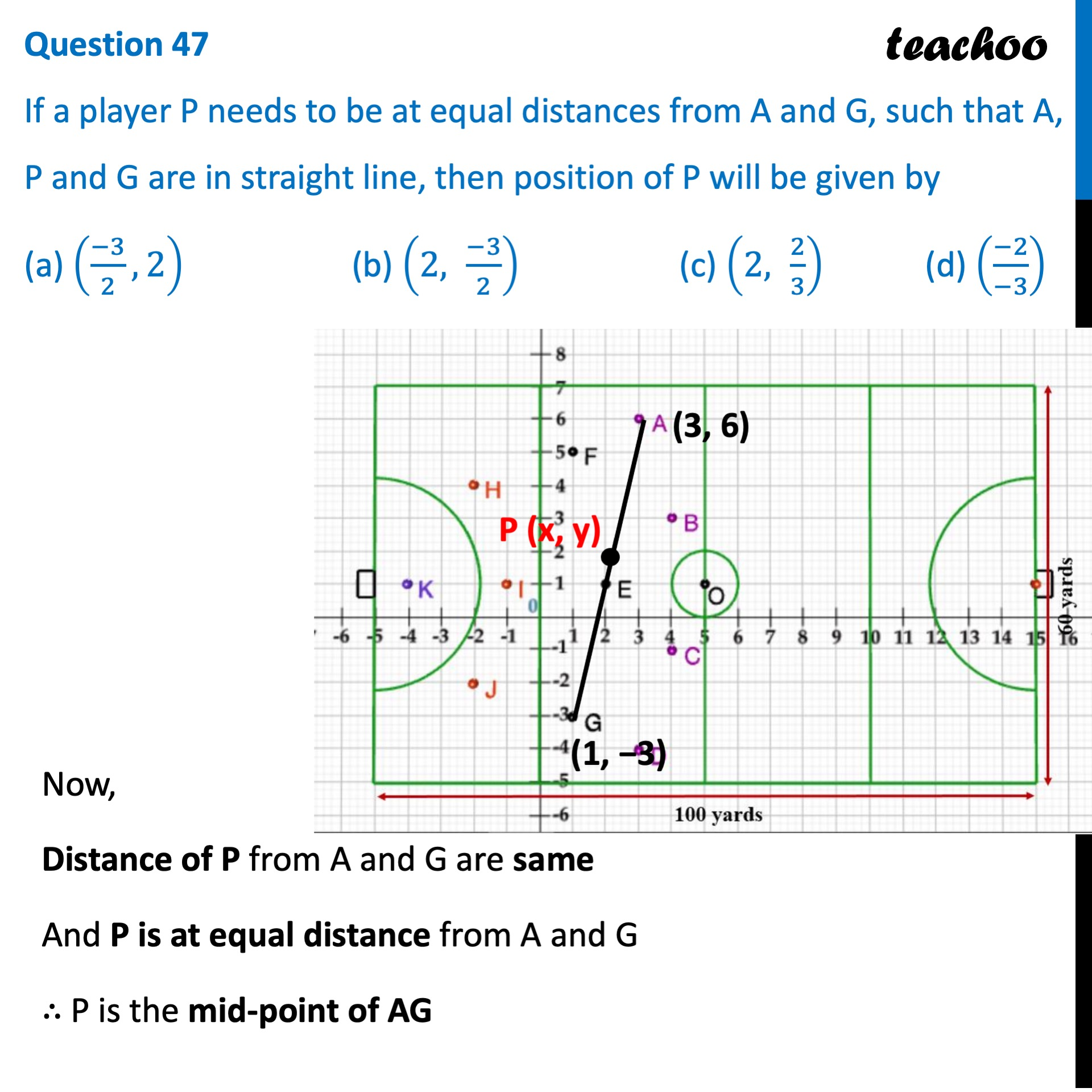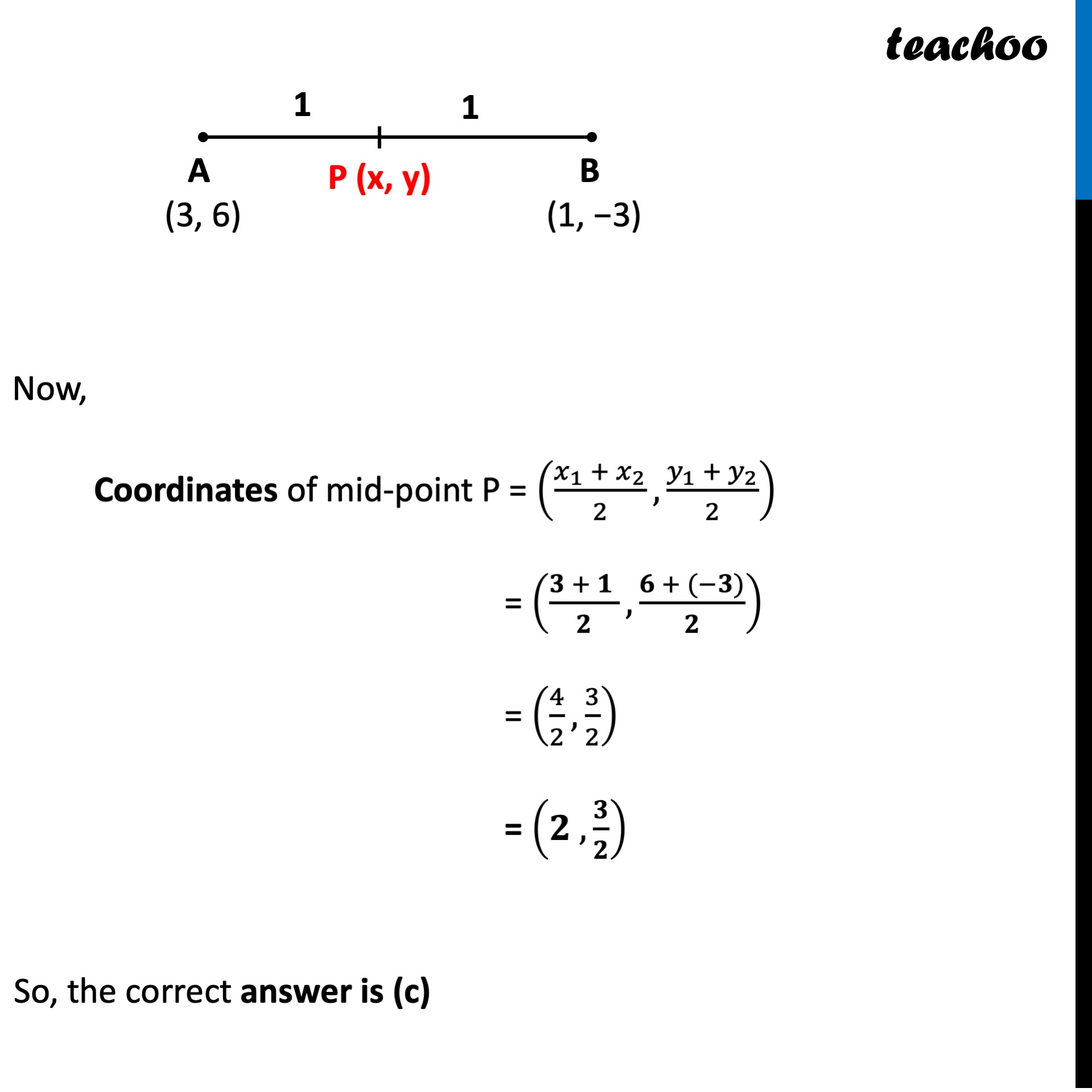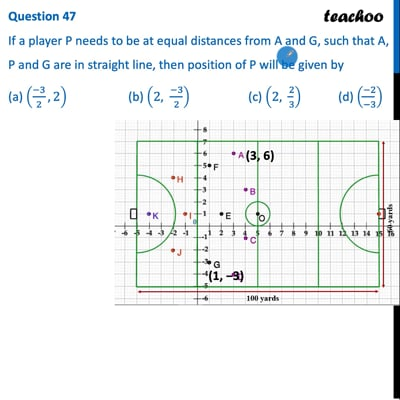CBSE Class 10 Sample Paper for 2022 Boards - Maths Standard [MCQ]

Class 10
Solutions of Sample Papers for Class 10 Boards

## (a) ((-3)/2, 2)  (b) (2,  (-3)/2)  (c) (2,  2/3)  (d) ((-2)/(-3))This video is only available for Teachoo black users

Introducing your new favourite teacher - Teachoo Black, at only ₹83 per month

### Transcript

Question 47 If a player P needs to be at equal distances from A and G, such that A, P and G are in straight line, then position of P will be given by (a) ((−3)/2, 2) (b) (2, (−3)/2) (c) (2, 2/3) (d) ((−2)/(−3)) Now, Distance of P from A and G are same And P is at equal distance from A and G ∴ P is the mid-point of AG Now, Coordinates of mid-point P = ((𝑥_1 + 𝑥_2)/2,(𝑦_1 + 𝑦_2)/2) = ((𝟑 + 𝟏 )/𝟐,(𝟔 + (−𝟑))/𝟐) = (4/2,3/2) = (𝟐 ,𝟑/𝟐) So, the correct answer is (c)